Courses

# Newton's Interpolation - Interpolation and Extrapolation, Business Mathematics and Statistics B Com Notes | EduRev

## B Com : Newton's Interpolation - Interpolation and Extrapolation, Business Mathematics and Statistics B Com Notes | EduRev

The document Newton's Interpolation - Interpolation and Extrapolation, Business Mathematics and Statistics B Com Notes | EduRev is a part of the B Com Course Business Mathematics and Statistics.
All you need of B Com at this link: B Com

Newton's Interpolation Formulae

As stated earlier, interpolation is the process of approximating a given function, whose values are known at N + 1 tabular points, by a suitable polynomial, PN(x), of degree N which takes the values yi at x = xi for i = 0, 1,,...,N. Note that if the given data has errors, it will also be reflected in the polynomial so obtained.

In the following, we shall use forward and backward differences to obtain polynomial function approximating  y = f(x), when the tabular points xi 's are equally spaced. Let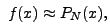where the polynomial P(x) is given in the following form:

P(x)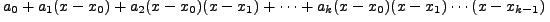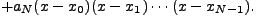(11.4.1)

for some constants a0 , a1, .... ato be determined using the fact that PN (xi) = yi for i = 0,1,...,N

So, for i = o substitute x = x0 in (11.4.1) to get  PN (x0) = yThis gives us a0 = yNext,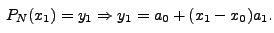So,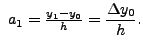For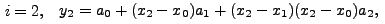or equivalently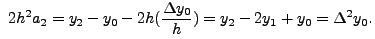Thus,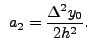Now, using mathematical induction, we get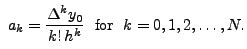Thus,

PN (x) =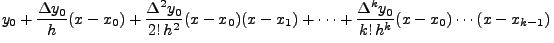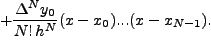As this uses the forward differences, it is called NEWTON'S FORWARD DIFFERENCE FORMULA for interpolation, or simply, forward interpolation formula.

EXERCISE 11.4.1   Show that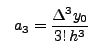and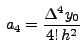and in general,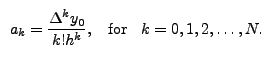For the sake of numerical calculations, we give below a convenient form of the forward interpolation formula.

Let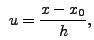then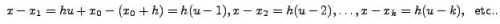With this transformation the above forward interpolation formula is simplified to the following form:

PN (u) =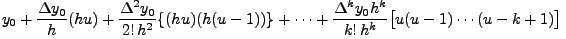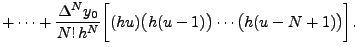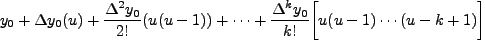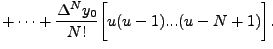(11.4.2)

If N =1, we have a linear interpolation given by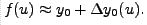(11.4.3)

For N = 2, we get a quadratic interpolating polynomial: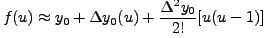(11.4.4)

and so on.

It may be pointed out here that if f(x) is a polynomial function of degree N then PN (x) coincides with f(x) on the given interval. Otherwise, this gives only an approximation to the true values of f(x)

If we are given additional point xN+1 also, then the error, denoted by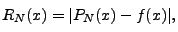is estimated by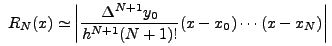Similarly, if we assume, PN(x) is of the form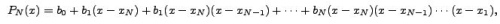then using the fact that PN (x= yi) we have

b= yN

b1 =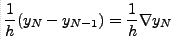b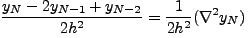.

.

.

b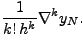Thus, using backward differences and the transformation  x= xN + hwe obtain the Newton's backward interpolation formula as follows: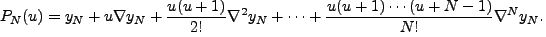(11.4.5)

EXERCISE 11.4.2   Derive the Newton's backward interpolation formula (11.4.5) for N = 3

Remark 11.4.3   If the interpolating point lies closer to the beginning of the interval then one uses the Newton's forward formula and if it lies towards the end of the interval then Newton's backward formula is used.

Remark 11.4.4   For a given set of n tabular points, in general, all the n points need not be used for interpolating polynomial. In fact N is so chosen that Nth forward/backward difference almost remains constant. Thus N is less than or equal to n.

EXAMPLE 11.4.5

1. Obtain the Newton's forward interpolating polynomial, P(x) for the following tabular data and interpolate the value of the function at x = 0.0045.x

 x 0 0.001 0.002 0.003 0.004 0.005 y 1.121 1.123 1.1255 1.127 1.128 1.1285

Solution: For this data, we have the Forward difference difference table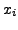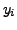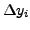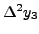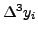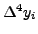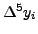0 1.121 0.002 0.0005 -0.0015 0.002 -.0025 .001 1.123 0.0025 -0.0010 0.0005 -0.0005 .002 1.1255 0.0015 -0.0005 0.0 .003 1.127 0.001 -0.0005 .004 1.128 0.0005 .005 1.1285

Thus, for  x = x+ hu, where  x0 = 0, h = 0.001 and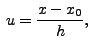we get

P5(x) =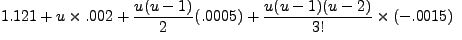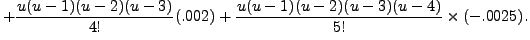Thus,

P5(0.0045) = P5 (0.001x4.5)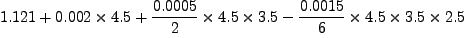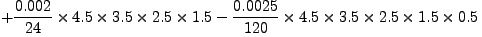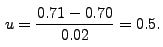= 1.12840045.

2. Using the following table for  tan x, approximate its value at 0.71 Also, find an error estimate (Note  tan (0.71) = 0.85953).0.7 72 0.74 0.76 0.78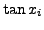0.84229 0.87707 0.91309 0.95045 0.98926

Solution: As the point x = 0.71 lies towards the initial tabular values, we shall use Newton's Forward formula. The forward difference table is: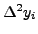0.70 0.84229 0.03478 0.00124 0.0001 0.00001 0.72 0.87707 0.03602 0.00134 0.00011 0.74 0.91309 0.03736 0.00145 0.76 0.95045 0.03881 0.78 0.98926

In the above table, we note that  Δ3y is almost constant, so we shall attempt 3rd degree polynomial interpolation.

Note that  x= 0.70, h = 0.02 givesThus, using forward interpolating polynomial of degree 3, we get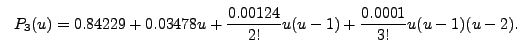Thus, tan(0.71) ≈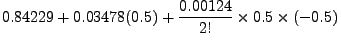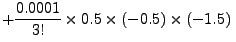= 0.859535

An error estimate for the approximate value is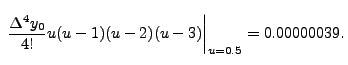Note that exact value of  tan (0.71) (upto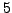decimal place) is 0.85953 and the approximate value, obtained using the Newton's interpolating polynomial is very close to this value. This is also reflected by the error estimate given above.

3. Apply 3rd degree interpolation polynomial for the set of values given in Example 11.2.15, to estimate the value of  f (10.3) by taking

(i) x0 = 9.0,    (ii) x= 10.0

Also, find approximate value of  f (13.5)

Solution: Note that x = 10.3x is closer to the values lying in the beginning of tabular values, while x =13.5 is towards the end of tabular values. Therefore, we shall use forward difference formula for x = 10.3 and the backward difference formula for x = 13.5 Recall that the interpolating polynomial of degree 3 is given by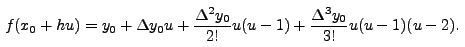Therefore,

1. for x0 = 9.0, h = 1.0 and x = 10.3, we have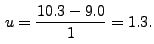This gives,

f (10.3) ≈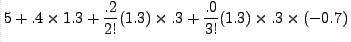= 5.559

2. for x0 = 10.0, h=1.0 and x = 10.3 we have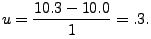This gives,

f(10.3)  ≈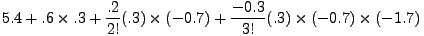= 5.54115.

Note: as x = 10.3x is closer to  x = 10.0, we may expect estimate calculated using x0 = 10.0 to be a better approximation.

1. for x0 = 13.5, we use the backward interpolating polynomial, which gives,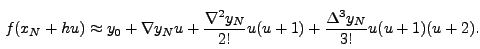Therefore, taking x= 14, h = 1.0 and  x = 13.5, we have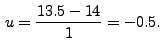This gives,
f(13.5) ≈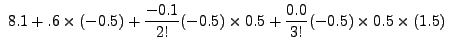= 7.8125

EXERCISE 11.4.6

1. Following data is available for a function y = f(x)

 x 0 0.2 0.4 0.6 0.8 1 y 1 0.808 0.664 0.616 0.712 1

Compute the value of the function at x = 0.3 and x = 1.1

2. The speed of a train, running between two station is measured at different distances from the starting station. If x is the distance in km from the starting station, then v (x) the speed (in km/hr ) of the train at the distance x is given by the following table:

 x 0 50 100 150 200 250 v(x) 0 60 80 110 90 0

Find the approximate speed of the train at the mid point between the two stations.

3. Following table gives the values of the function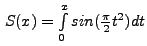at the different values of the tabular points x,

 x 0 0.04 0.08 0.12 0.16 0.2 S(x) 0 3e-05 0.00026 0.0009 0.00214 0.00419

Obtain a fifth degree interpolating polynomial for S(x) Compute S (0.02) and also find an error estimate for it.

4. Following data gives the temperatures (in 0C ) between 8.00 am to 8.00 pm. on May 10, 2005 in Kanpur:

 Time 8 am 12 noon 4 pm 8pm Temperature 30 37 43 38

Obtain Newton's backward interpolating polynomial of degree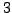to compute the temperature in Kanpur on that day at 5.00 pm.

Offer running on EduRev: Apply code STAYHOME200 to get INR 200 off on our premium plan EduRev Infinity!

122 videos|142 docs

,

,

,

,

,

,

,

,

,

,

,

,

,

,

,

,

,

,

,

,

,

,

,

,

;